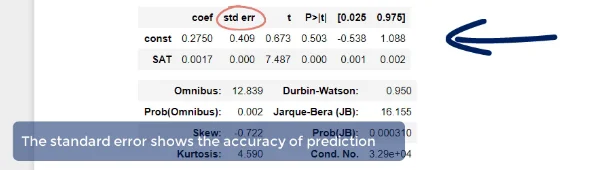Posted on:

28 Nov 2022

0

# If lower coef std error indicates better estimate, is there convention on interpreting std err value

Hello lovely people,

The video mentions that in the coefficients table, "The standard error shows the accuracy of prediction" and that the lower this is, the better the estimate (time after 3:00 mark).I'm wondering if there any sort of conventional wisdom on how to interpret these values? It makes sense that if it's smaller (closer to 0) then the estimate is better, but in practice this wouldn't be exactly 0 every time, right?

I also recalled that the data visualization course mentions that R squared is an example of a value that interpretation of what's "acceptable" can vary depending on the specific industry.

In summary, my questions are:
(1) Are these std err of coefficients something typically used with assessing accuracy of prediction and is there a quantifiable way to do so? Or is it used elsewhere in statistical analysis rather than just assessing the raw value?
(2) Does some sort of conventional wisdom/rule of thumb exist for assessing these std err of coefficients (i.e. values that indicate "can roughly tell this isn't acceptable/doesn't look right")

Let me know if you need any clarification on my questions! Thanks for the help :)